# Triangle Formula

A triangle is a polygon with three edges and three vertices. It is one of the basic shapes in geometry. A triangle with vertices A, B, and C.The length of the sides of a triangle may be same or different. If all the 3 sides of a triangle are equal then it is an equilateral triangle. If 2 sides of a triangle are equal, it is an isosceles triangle.

### Types of Triangles

Equilateral Triangles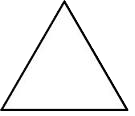The Equilateral Triangles have the following properties (in addition to the properties above for all triangles):

• Three straight sides of equal length
• Three angles, all equal to 60°
• Three lines of symmetry

Isosceles Triangles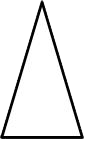The Isosceles Triangles have the following properties:

• Two sides of equal length
• Two equal angles
• One line of symmetry

Scalene Triangles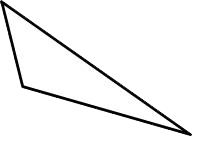Scalene triangles have the following properties

• No sides of equal length
• No equal angles
• No lines of symmetry

Acute trianglesAcute triangles have all acute angles (angles less than 90°). It is possible to have an acute triangle which is also an isosceles triangle – these are called acute isosceles triangles.

Right TrianglesThe Right Triangles (right-angled triangles) have one right angle (equal to 90°).It is possible to have a right isosceles triangle – a triangle with a right angle and two equal sides.

Obtuse trianglesObtuse triangles have one obtuse angle (angle which is greater than 90°). It is possible to have a obtuse isosceles triangle – a triangle with an obtuse angle and two equal sides.

The Triangle Formula are given below as,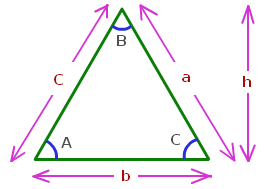Perimeter of a triangle = a + b + c

$Area\; of \; a\; triangle= \frac{1}{2}bh$

Where,
b is the base of the triangle.
h is the height of the triangle.

If only 2 sides and an internal angle is given then the remaining sides and angles can be calculated using the below formula:

$\large \frac{a}{sinA}=\frac{b}{sinB}=\frac{c}{sinC}$

### Solved Examples Using Triangle Formula

Example: Given the following information: c = 8, b = 12, C = 30°

Note that we are given two side lengths and an angle which is not the included angle. Referring back to the special cases described in the Introduction you will see that with this information there is the possibility that we can obtain two distinct triangles with this information.

As before we need a sketch in order to understand the information.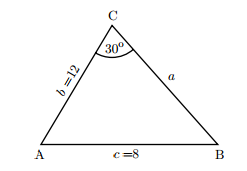The above diagram we are given two sides and a non-included angle. Because we have been given two sides and a non-included angle we use the sine formulae.

$$\begin{array}{l}\frac{a}{sinA}=\frac{b}{sinB}=\frac{c}{sinC}\end{array}$$

Or

$$\begin{array}{l}\frac{sinA}{a}=\frac{sinB}{b}=\frac{sinC}{c}\end{array}$$

Because we are given b, c and C we use the following part of the formula in order to find angle B.

$$\begin{array}{l}\frac{sinB}{b}=\frac{sinC}{c}\end{array}$$

$$\begin{array}{l}\frac{sinB}{12}=\frac{sin\, 30^{\circ}}{8}\end{array}$$

$$\begin{array}{l}sin\,B=\frac{12\times sin\, 30^{\circ}}{8}\end{array}$$

$$\begin{array}{l}=\frac{12\times \frac{1}{2}}{8}\end{array}$$

$$\begin{array}{l}=\frac{6}{8}\end{array}$$

$$\begin{array}{l}=\frac{3}{4}\end{array}$$

=0.75

$$\begin{array}{l}B=sin^{-1}\; 0.75\end{array}$$

=

$$\begin{array}{l}48.6^{\circ}\end{array}$$
(1 d.p.)

Now there is a potential complication here because there is another angle with sine equal to 0.75. Specifically, B would equal

$$\begin{array}{l}180^{\circ}\end{array}$$
−
$$\begin{array}{l}48.6^{\circ}\end{array}$$
=
$$\begin{array}{l}131.4^{\circ}\end{array}$$
.

In the first case the angles of the triangle are then:

C =

$$\begin{array}{l}30^{\circ}\end{array}$$
, B =
$$\begin{array}{l}48.6^{\circ}\end{array}$$
, A =
$$\begin{array}{l}180^{\circ}\end{array}$$
−
$$\begin{array}{l}78.6^{\circ}\end{array}$$
=
$$\begin{array}{l}101.4^{\circ}\end{array}$$

In the second case we have:

C =

$$\begin{array}{l}30^{\circ}\end{array}$$
, B =
$$\begin{array}{l}131.4^{\circ}\end{array}$$
, A =
$$\begin{array}{l}180^{\circ}\end{array}$$
−
$$\begin{array}{l}161.4^{\circ}\end{array}$$
=
$$\begin{array}{l}18.6^{\circ}\end{array}$$
.

The situation is depicted in the the figure below. In order to solve the triangle completely we must deal with the two cases separately in order to find the remaining unknown a.

Case 1. Here C =

$$\begin{array}{l}30^{\circ}\end{array}$$
, B =
$$\begin{array}{l}48.6^{\circ}\end{array}$$
, A =
$$\begin{array}{l}101.4^{\circ}\end{array}$$
. We use the sine rule in the form

$$\begin{array}{l}\frac{a}{sin\, A}=\frac{b}{sin\, B}\end{array}$$

from which

$$\begin{array}{l}a=\frac{12sin\, 101.4^{\circ}}{sin\,48.6^{\circ}}\end{array}$$

= 15.7 (1 d.p.)

Case 2. Here C =

$$\begin{array}{l}30^{\circ}\end{array}$$
, B =
$$\begin{array}{l}131.4^{\circ}\end{array}$$
, A =
$$\begin{array}{l}18.6^{\circ}\end{array}$$
. Again we can use the sine rule in the form

$$\begin{array}{l}\frac{a}{sin\, A}=\frac{b}{sin\, B}\end{array}$$

from which

$$\begin{array}{l}a=\frac{12sin\, 18.6^{\circ}}{sin\,131.4^{\circ}}\end{array}$$

= 5.1 (1 d.p.)